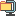검색어 입력폼

# Chapter 3. BJT Amplifier Design Experiments

저작시기 2014.12 | 등록일 2015.02.07압축파일 (zip) | 6페이지 | 가격 1,000원

## 목차

1. Determine the voltage gain of the circuit shown in Fig 3.3
2. What is the means of “Bandwidth(BW)” at the amplifier?
3. PSPICE practice – Design the circuit shown in Fig 3.4, Determine the voltage Gain and BW. Also, compute Input/output waveform. (Input Amplitude = 1mV)
4. Explain about the reason that restrains the Bandwidth of the circuit shown in Fig 3.4 (Ref. Fundamentals of Microelectronics, Behzad Razavi, pp. 564~572)
5. To maximize Gain-Bandwidth product(voltage gain * BW[kHz] / Power[mW]) through the R7 & R6 shown in Fig 3.4.

## 본문내용

What is the means of “Bandwidth(BW)” at the amplifier?
To discuss the bandwidth, we need to know about “roll-off” factor. “Roll-off” factor happens when the amplifier meets some large enough frequency. All the circuits include the node, and node actually has its capacitance. These actual capacitors form the pole in the circuit. The pole has property that decreases the voltage gain in the amplifier.

## 참고 자료

Wikipedia, Fundamentals of Microelectronics, Behzad Razavi

3주차 결과.docx
3주차 예비.docx